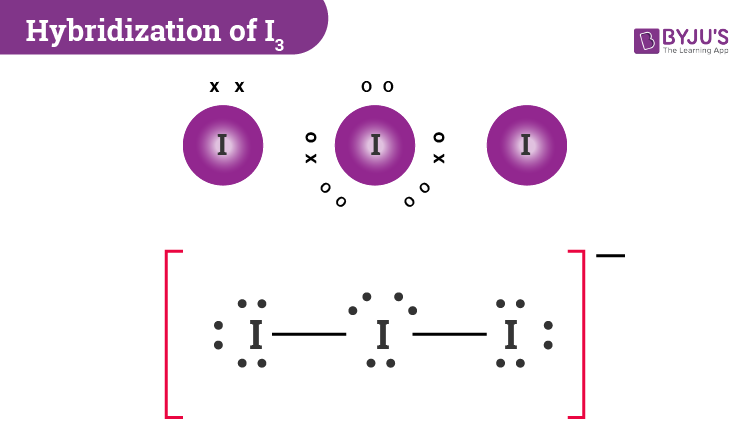# Hybridization of I3(-)

Here we will learn how to determine the hybridization of I3-. One key thing that students have to remember is that I3− is a linear anion. It is formed by the bonding of I2 with I− ion. Here I− ion will be the donor and I2 molecule will be the acceptor where electrons are usually accommodated in the empty d orbitals. I3- ion is basically sp3d hybridized.

 Name of the Molecule Triiodide ion Molecular Formula I3- Hybridization Type sp3d Bond Angle 180o Geometry Linear

## What is the Hybridization of Triiodide ion?

To know the hybridization of Triiodide ion, we can use simple hybridization formula which is given as;

 Number of Hybridization = Valence electron + monovalent + (negative charge) – (positive charge)/2

If we look at the iodine atoms there are seven valence electrons in its outer shell and two monovalent atoms are also present. Further, during the combination of Iodine with the two other Iodine atoms, the central atom gains a negative charge whose value will be taken as 1.

If we place or substitute the values according to the formula, we get

7+1+2/2

=10/2

=5

Therefore the hybridisation number is equal to 5. Now we can say that hybridisation is sp3d.

Alternatively, we can also determine the hybridization of I3- by knowing the number of valence electrons and lone pairs and calculating their sum. In this case, if we consider the lone pairs there are 3 such pairs while the number of atoms donating valence electrons is 2. If we add these values together we get 5 which can be interpreted as sp3d hybridisation.

### Important Points To Remember

• I3- is formed by the bonding of I2 with I− ion.
• During the combination of Iodine atoms, the central atom gains a negative charge whose value will be 1.
• I− ion is the donor and I2 molecule is the acceptor. Electrons are mostly accommodated in the empty d orbitals.

## I3- Molecular Geometry And Bond AnglesI3- molecular geometry is linear. While there are three Iodine atoms, one of the atoms has a negative charge which further gives 3 lone pairs of electrons and 2 bond pairs. Its steric number will be 5. The three lone pairs will repel each other and take up equatorial positions. The remaining two Iodine atoms are at 180o from each other.